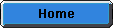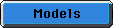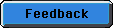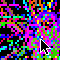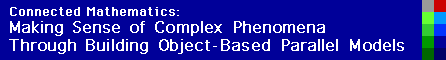Binomial Rabbits

This model simulates rabbits hopping in a one-dimensional space (a line). This model was created by a student in order to undersatand how normal distributions can emerge from simple probabilistic rules. He used the model to explain why it might come to be that height is distributed normally.

To run the model, the user specifies the following parameters:

• The number of rabbits in the simulation,
• The direction and distance of each of the different possible "hops" that each rabbit may make in a turn, and
• The probability that a rabbit makes each particuar "hop."

Each of the rabbits makes one hop per turn. After each turn, the program updates its ouput window to show a graph of the current positions of the rabbits relative to their starting position. The user may adance the simulation one turn at a time, or let the simulation run continuously so as to view the gradual movement of the rabbits over many turns.

For detailed instructions on using the program, take a look at the info window from the Binomial Rabbits model.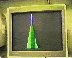Equal Probabilities, Equal Step Sizes. (2.9 M)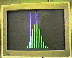Equal Probabilities, Unequal Step Sizes. (3 M)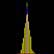Rabbits : This model demonstrates 1000 rabbits hopping one step to the left and one step to the right, with equal probability for 25 hops. (180 k)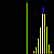Rabbits : This model demonstrates 1000 rabbits hopping one step to the left and three steps to the right, and are three times more likely to move to the right than the left for 15 hops. (111 k)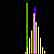Rabbits : This model demonstrates 1000 rabbits hopping one step to the left and three steps to the right, with equal probability for 15 hops. (115 k)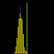Rabbits : This model demonstrates 1000 rabbits hopping one step to the right and one step to the left, and are three times more likely to move to the left than the right for 25 hops. (178 k)